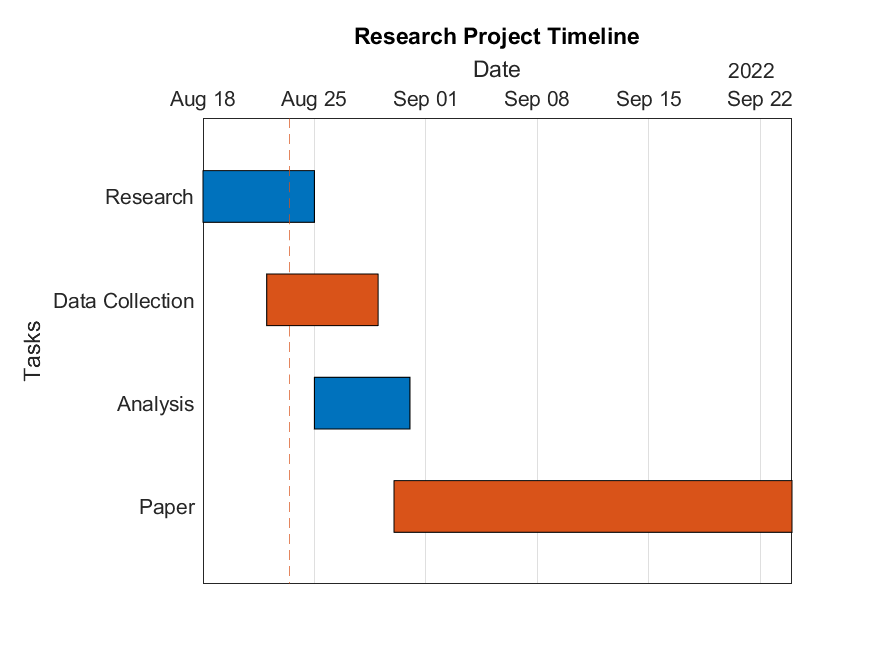## Gantt Chart

Version 1.0 (318 KB) by
Visualizes the start and end dates of various tasks in a project.

Updated 30 Aug 2022

From GitHub

# Gantt ChartVersion: 1.0

Visualizes the start and end dates of various tasks in a project.## Syntax

Note: By default, events are arranged vertically and are ordered based on the order of the input.

• `ganttChart(TaskData, DurationData)` - creates a bar for each task. The length of the bar represents the duration of the respective task. Each task's start date is the previous task's end date. The first task starts at the current time.
• `ganttChart(TaskData, StartDate, EndDate)` - creates a bar for each task as described above. The bar's length corresponds to the duration between a given start and end date.
• `ganttChart(TaskData, StartDate, DurationData)` - creates a bar for each task. The end date is calculated by adding a given duration to the respective start date.
• `ganttChart(TaskData, DurationData, EndDate)` - creates a bar for each task. The start dates of the tasks are calculated by subtracting the duration from the task's end date, to find the latest start date.
• `ganttChart(_, Name, Value)` - specifies additional options for the chart using one or more name-value pair arguments. Specify the options after all other input arguments.
• `ganttChart(target, _)` - plots into target instead of GCF.
• `f = ganttChart(_)`- returns the ganttChart object. Use `f` to modify properties of the chart after creating it.

This information is also available if you call `help ganttChart`.

## Name-Value Pair Arguments/Properties

• `TaskData` - (1 x n text vector) the task data containing n tasks.
• `DurationData` - (1 x n duration vector) the time duration of each n task.
• `StartDate` - (1 x n datetime vector) the start date of each n task.
• `Title` - (1 x n char vector) title of the Gantt chart.
• `TaskAxisLabel` - (1 x n text vector) y-label of the task axis.
• `TimeAxisLabel` - (1 x n text vector) x-label of the time axis.
• `FaceColor` - (N x 3 RGB triplets or N x 1 hexadecimal color codes where N ≥ 1) the fill color of each bar. Note that color wrapping occurs when N < n by default.
• `EdgeColor` - (1 x 3 RGB triplet or a hexadecimal color code) the outline color of each bar.
• `Grid` ('on', 'off', or 'columns') - specifies which major grid lines are visible.
• `BarWidth` - (double ≤ 1) the width of each bar.
• `LineWidth` - (double) the width of each edge of a bar.
• `ShowNowLine` - (scalar `matlab.lang.OnOffSwitchState`) specifies whether to display the 'now line', a vertical line at the current datetime of when `ganttChart(_)` was called.
• `NowLineColor` - (1 x 3 RGB triplet) the color of the now line.
• `NowLineStyle` - ('-', '--', ':', or '-.') the line style of the now line.

## Methods

• `sort(obj, order)` - sorts the tasks by start date in ascending or descending order.
• `addTask(obj, task, dur, startDate)` - adds a new task to the existing Gantt chart. The task is added at the bottom of the chart in an unsorted order.
• `stagger(obj, taskOne, taskTwo)` - staggers two overlapping tasks by shifting one down. Note that if two tasks in `TaskData` have the same name, this method will only stagger the first matching task.

## Example

Run the `example.mlx` file to view more examples.

Initialize the data below containing various research tasks.

``````Task = ["Research"
"Data Collection"
"Analysis"
"Paper"];

StartDate = [datetime(2022,4,20)
datetime(2022,4,24)
datetime(2022,5,1)
datetime(2022,5,7)];

EndDate = [datetime(2022,4,27)
datetime(2022,5,1)
datetime(2022,5,7)
datetime(2022,6,1)];

Duration = days([7; 7; 6; 25]);

datetime(2022, 5, 16)
datetime(2022, 4, 29)
datetime(2022, 5, 21)];
``````

Plot the Gantt chart and label the axes.

``````g = ganttChart(Task, Duration);
g.Title = 'Research Project Timeline (Sample Gantt Chart)';
g.TimeAxisLabel = 'Date';
g.FaceColor = ['#0072BD'; '#D95319'];
``````

### Cite As

Alexis Wang (2023). Gantt Chart (https://github.com/MATLAB-Graphics-and-App-Building/gantt-chart/releases/tag/v1.0), GitHub. Retrieved .

##### MATLAB Release Compatibility
Created with R2022a
Compatible with R2022a
##### Platform Compatibility
Windows macOS Linux